# Using

Using the point-slope equation, find the equation containing (-7, 3) and slope m = -4

Result

f = (Correct answer is: -4x-25)### Step-by-step explanation: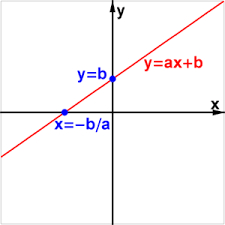Did you find an error or inaccuracy? Feel free to write us. Thank you!Tips to related online calculators
Line slope calculator is helpful for basic calculations in analytic geometry. The coordinates of two points in the plane calculate slope, normal and parametric line equation(s), slope, directional angle, direction vector, the length of the segment, intersections of the coordinate axes, etc.

## Related math problems and questions:

• V - slopeThe slope of the line whose equation is -3x -9 = 0 is
• Slope formFind the equation of a line given the point X(8, 1) and slope -2.8. Arrange your answer in the form y = ax + b, where a, b are the constants.
• Perpendicular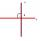Determine the slope of the line perpendicular to the line p: y = -x +4.
• Slope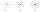Calculate the slope of a line that intersects points (-84,41) and (-76,-32).
• SlopeWhat is the slope of the line defined by the equation -2x +3y = -1 ?
• Three points 4The line passed through three points - see table: x y -6 4 -4 3 -2 2 Write line equation in y=mx+b form
• Line segmentFor the line segment whose endpoints are L[-1, 13] and M[18, 2], find the x and y value for the point located 4 over 7 the distance from L to M.
• Points OPQ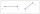Point P is on line segment OQ. Given OP = 6, OQ = 4x - 3, and PQ = 3x, find the numerical length of OQ.
• Midpoint 4If the midpoint of a segment is (6,3) and the other end point is (8,-4) what are thw coordinate of the other end?
• General line equationsIn all examples, write the GENERAL EQUATION OF a line that is given in some way. A) the line is given parametrically: x = - 4 + 2p, y = 2 - 3p B) the line is given by the slope form: y = 3x - 1 C) the line is given by two points: A [3; -3], B [-5; 2] D) t
• SlopeFind the slope of the line: x=t and y=1+t.
• Slope RR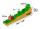A line has a rise of 2 and a run of 11. What is the slope?
• LineWrite an equation of a line parallel to To 9x + 3y = 8 That Passes Through The Point (-1, -4). Write in form ax+by=c.
• SlopeWhat is the slope of a line with an inclination 6.06 rad?
• Coordinates of midpointIf the midpoint of the segment is (6,3) and the other end is (8,4) what are the coordinate of the other end?
• Find the 3Find the distance and midpoint between A(1,2) and B(5,5).
• Perpendicular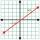What is the slope of the perpendicular bisector of line segment AB if A[9,9] and B[9,-2]?